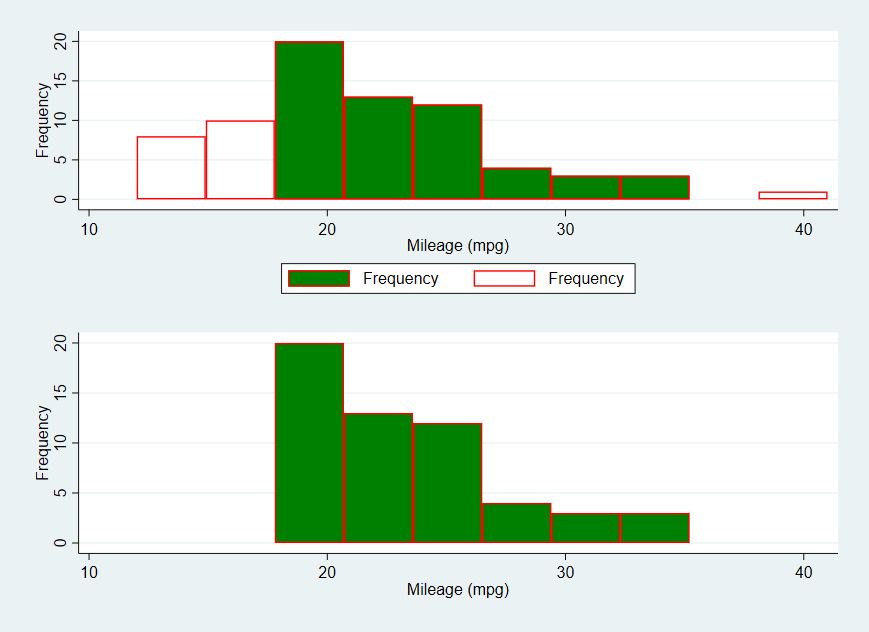Search

Displaying part of the histogram

Below is a worked example. The data set I used is auto.dta:The commands I used in a do-file are:

sysuse auto, clear

twoway__histogram_gen mpg , gen(h y, replace) freq bin(10)

twoway ///
(histogram y [fw=h] if inrange(y,19,35),discrete color(green)lcolor(red)freq) ///
(histogram y [fw=h],color(none) lcolor(red) discrete freq name(a1, replace))

twoway ///
(histogram y [fw=h] if inrange(y,19,35),discrete color(green) lcolor(red)freq ///
name(a2, replace))

graph combine a1 a2 ,xcommon cols(1)

For more information on Stata graphs, the book A Visual Guide to Stata Graphics, 3rd Edition is a very useful and handy book.